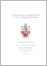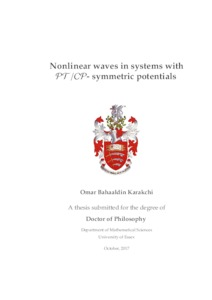# Nonlinear waves in systems with PT/CP - symmetric potentials

Karakchi, Omar Bahaaldin (2017) Nonlinear waves in systems with PT/CP - symmetric potentials. PhD thesis, University of Essex.Preview
Text
thesis_Omar Karakchi.pdf

Download (8MB) | Preview

## Abstract

Arguably, one of the most elementary nonlinear lattice dynamical models is the discrete nonlinear Schrödinger (DNLS) equation . Particularly, the discrete system and lattice solitons are the main objects of investigation in this work. In this thesis, we consider a chain of dimers, that is modelled by linearly coupled DNLS equations with gain and loss terms as analogues of Parity-Time (PT) symmetric systems. We construct fundamental bright discrete solitons of the systems and explore their spectral stability. The perturbation theory is used to perform the analysis in the case of weak coupling between the lattices, which is then verified by numerical calculations. Such analysis is based on the concept of the so-called anticontinuum limit approach. Also we consider an array of dual-core waveguides (which represent an optical realisation of a chain of dimers) with an active (gain-loss) coupling between the cores, opposite signs of the discrete diffraction in the parallel arrays, and a sufficiently large phase-velocity mismatch between them, which is necessary for the overall stability of the system. The corresponding linear array provides an optical emulation of the Charge-Parity (CP) symmetry. The addition of the intra-core cubic nonlinearity, despite breaking the CP-symmetry, gives rise to several families of fundamental bright discrete solitons, whose existence and stability are explored here by means of analytical and numerical methods. We study localised solutions in a (PT)-symmetric coupler composed by a chain of dimers, that is modelled by linearly coupled DNLS equations with gain and loss terms and with a cubic-quintic nonlinearity. We consider site-centered and bond-centered localised solutions and show that the resulting bifurcation diagrams when a parameter is varied form a snaking behaviour. Each localised solution has symmetric and antisymmetric configurations between the arms. We analyse the width of the snaking region and provide asymptotic approximations in the limit of strong and weak coupling where good agreement is obtained.

Item Type: Thesis (PhD) Q Science > QA Mathematics Faculty of Science and Health > Mathematical Sciences, Department of Omar Karakchi 27 Oct 2017 09:24 27 Oct 2017 09:24 http://repository.essex.ac.uk/id/eprint/20551

### Actions (login required)View Item